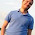## Friday, June 01, 2012

### Minimum language complexity needed to construct exponential time algorithm

(while this could have been a question on cstheory, I think it's a little too vague - but it's perfect for a blog post!)

I was at dinner with some faculty colleagues (assistant professor beer night - best invention known to mankind!) and we were in the traditional "all our students are terrible" phase of the evening *. The discussion turned to "what programming concepts do students understand after an intro-CS class", which led to the following claim:
"you can't write an exponential time algorithm without knowing recursion"
While this seemed plausible,  I was skeptical. Thankfully my PL colleague (and super blogger) Matt Might was at the table, and he pointed out that the lambda calculus formally has no recursive constructs and yet is Turing-complete, via the magic of the Y-combinator (which essentially computes fixed points directly).

Of course one might argue that a fixed point operator does give you recursion (indeed the Y-combinator is often referred to as anonymous recursion). So my question really is:

Is there a well defined notion of "programming languages without recursion or recursion-like substances" and if so, what is the most expensive algorithm that can be expressed in such a language ?

p.s BDDs seem like an obvious candidate...

1 (other regularly discussed phases include "I can't believe < latest cryptic thing > senior faculty member said", "How many frequent flyer miles do you have" and "chickens!". Beer night is awesome !

1.Interesting thoughts. I found this blog post, which claims "Even if we restrict ourselves to SQL-92, it is possible to write queries of polynomial, or even exponential complexity". Though there is no example query. The interesting point is that SQL-92 is not Turing-complete, but has primitive recursion.

2.Not even close. The standard iterative algorithm for the Tower of Hanoi puzzle takes exponential time.

Here's the algorithm:
(1) Move the only disk you can that you didn't just move.
(2) If the index of the disk is odd, move it clockwise; if it's even, move it counterclockwise.

3.ah excellent. Nicely exploits the structure of the problem to unroll the recursion compactly.

4.for i = 1 to 2^n: print "this is taking a long time'

Or, when you forbid "recursion", are you really forbidding looping? (See: http://en.wikipedia.org/wiki/Structured_program_theorem)

5.Dynamic programming is an important technique in exponential time algorithms. You might argue this actually involves recursion. But while the proof of correctness usually does involve induction, the reasonable implementation is often to iteratively fill a table.

6.I guess you may have intended to ask for "sane" computing models, but I get two associations to esoteric languages.

First, I wonder what the longest-running non-infinite n-step program in SMETANA is.

Second, there's SMITH (a related language), where you have no jumps or other flow control, except to copy selected part of your own program code to some place in front of the program counter. (Turing complete.)

7.Dynamic programming is an important technique in exponential time algorithms. You might argue this actually involves recursion. But while the proof of correctness usually does involve induction, the reasonable implementation is often to iteratively fill a table.

8.The language BlooP (Hofstadter) is a language that captures all primitive recursive sets (a proper subset of the recursive sets) without using recursion.

9.function(N):
for i from 1 to exp(N)
do something constant

10.Give me an array of booleans of length n, and I can simulate counting from 0 to 2^n, and also enumerate the power set of a size n input set. All with two loops and an if.

I think this was also the point of the LOOP programming language by Meyer and Ritchie, although they allowed recursion, and so could compute all primitive recursive functions.

11.I'm not sure if there's any relation between exponential time algorithms and recursion except for the fact that there are some recursive algorithms that take exponential time.

For example, the naive algorithm to decide if a number is prime. It iterates over all numbers from 1 to n and checks if they divide n.

Along similar lines, any algorithm that iterates over all subsets of a set provided as the input will take exponential time.

12.input: n

sum = 1
for i from 1 to n:
sum = 2 * sum
for i from 1 to sum:
// do stuff

// n + 2^n much work has been done.

13.The Grzegorczyk Hierarchy:

Starting just with X<-0 and X <- X+1 and simple nested for loops you can get each level of the Ackermann function. In particular exponential time requires only 2 nested loops.

However, the hierarchy DOES show that there is a limit without either recursion or unbounded looping, namely you can't get to non-elementary functions like Ackermann's function itself.

14.Maybe the point should be that almost any recursive algorithm an undergraduate writes will be exponential time.

15.Well, WHILE programs (afaik a standard model of computation) are Turing complete and don't provide recursion, so you can obviously express exponential (and worse) algorithms in it.

16.I suggest that you look at type lambda calculi  that correspond to constructive logics via the Curry-Howard correspondence . They do not allow to type the Y-combinator and other related combinators. They generally enables us express superexponential algorithms, and there's a rich theory of exactly what can be expressed in these calculi.

 http://www.rbjones.com/rbjpub/logic/cl/tlc001.htm
 http://en.wikipedia.org/wiki/Curry%E2%80%93Howard_correspondence